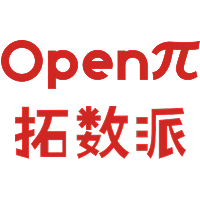# 数据科学在量化金融中的应用：指数预测（上）2022-12-29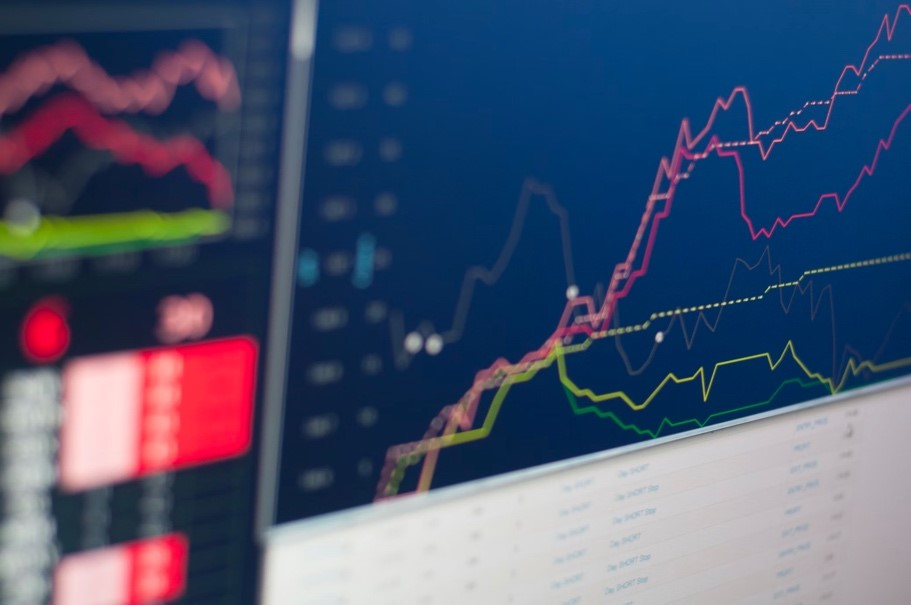• 确定预测目标
• 数据收集
• 探索性数据分析
• 数据预处理
• 特征工程
• 模型选择和训练
• 模型评估
• 模型预测

## 数据收集

```import yfinance as yf
```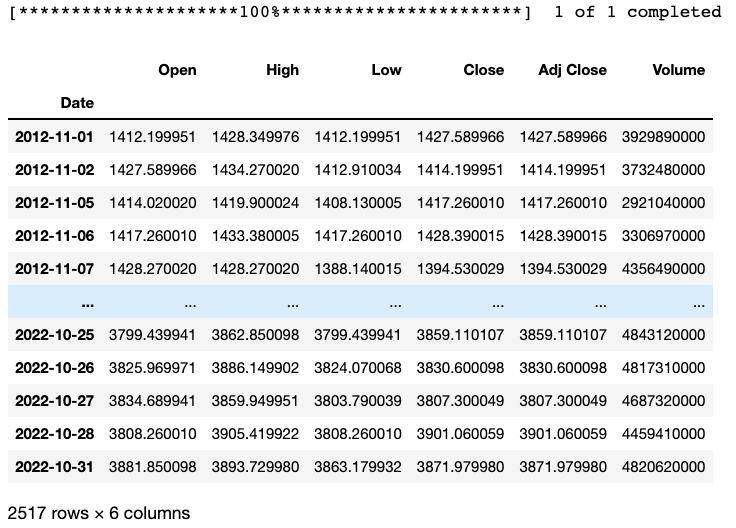Pandas数据集“df_sp”包含了从2012年11月1日至2022年10月31日十年以来的标普500指数数据，记录了每个日期所对应的开市价格、最高价格、最低价格、闭市价格、闭市调整价格和交易量。

## 探索性数据分析

```df_sp.info()
```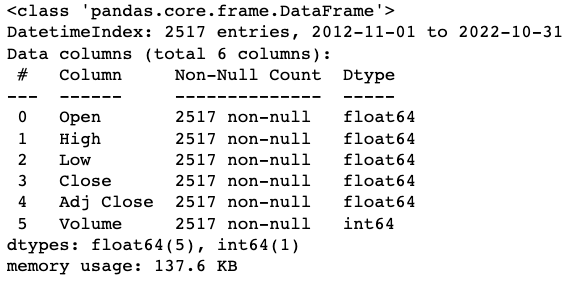```df_sp.describe()
```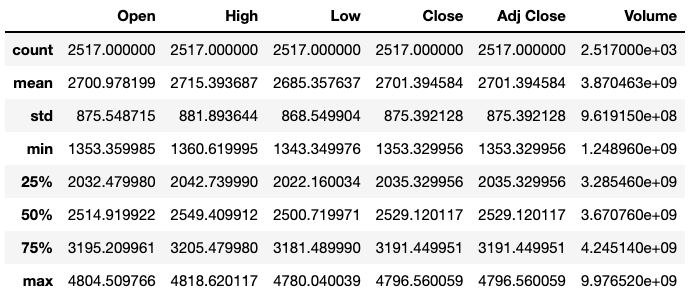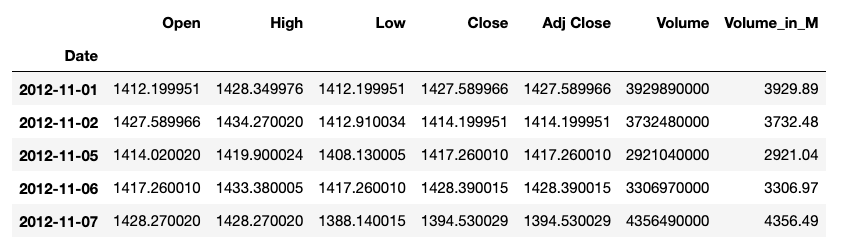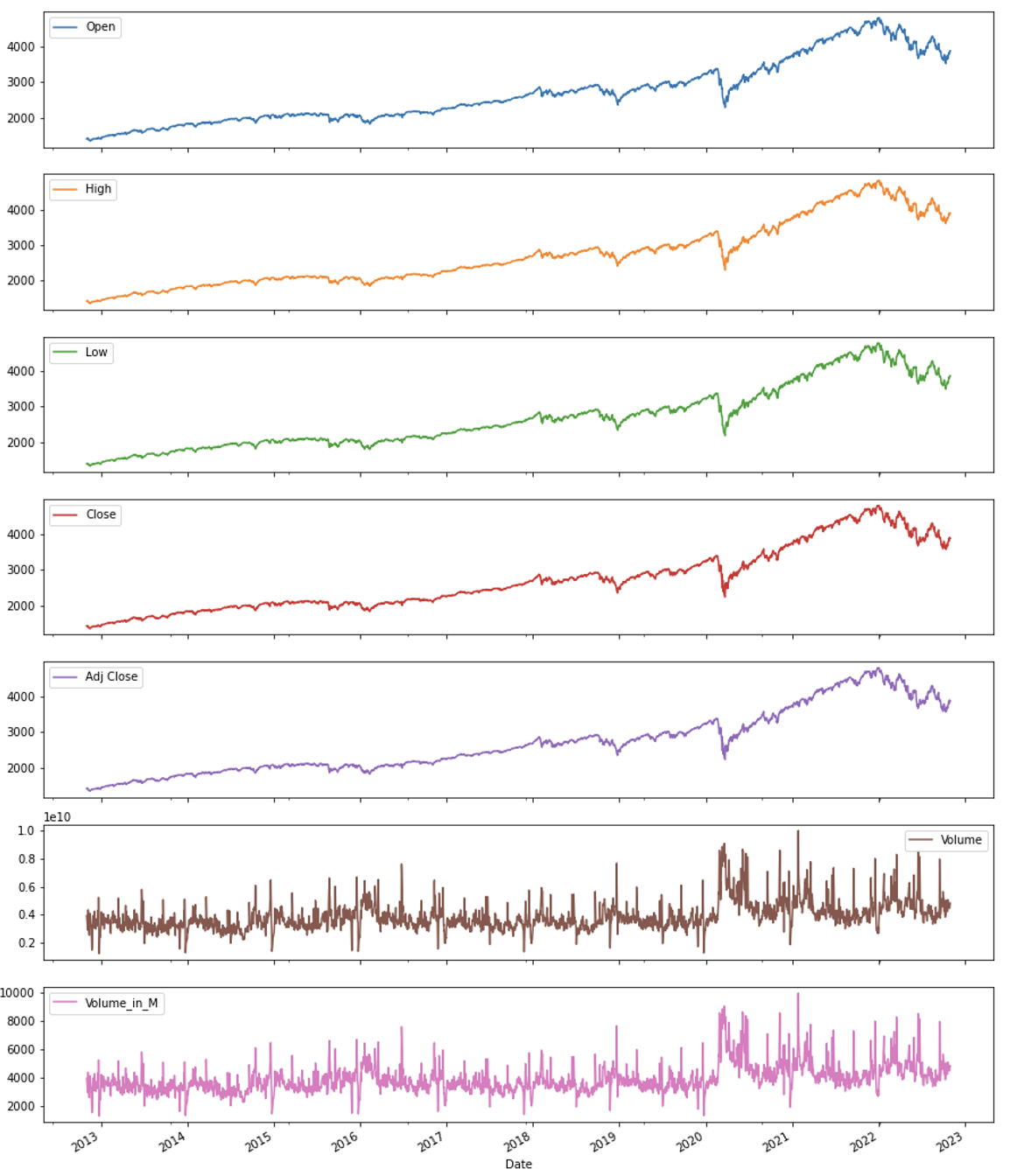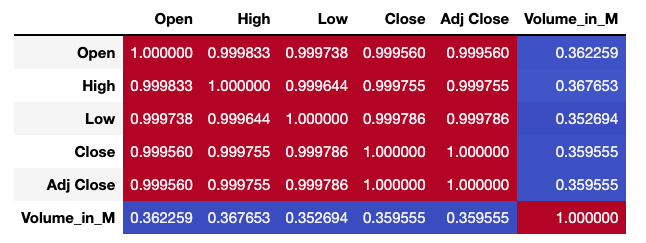```df = df_sp[['Adj Close','Volume_in_M']]
```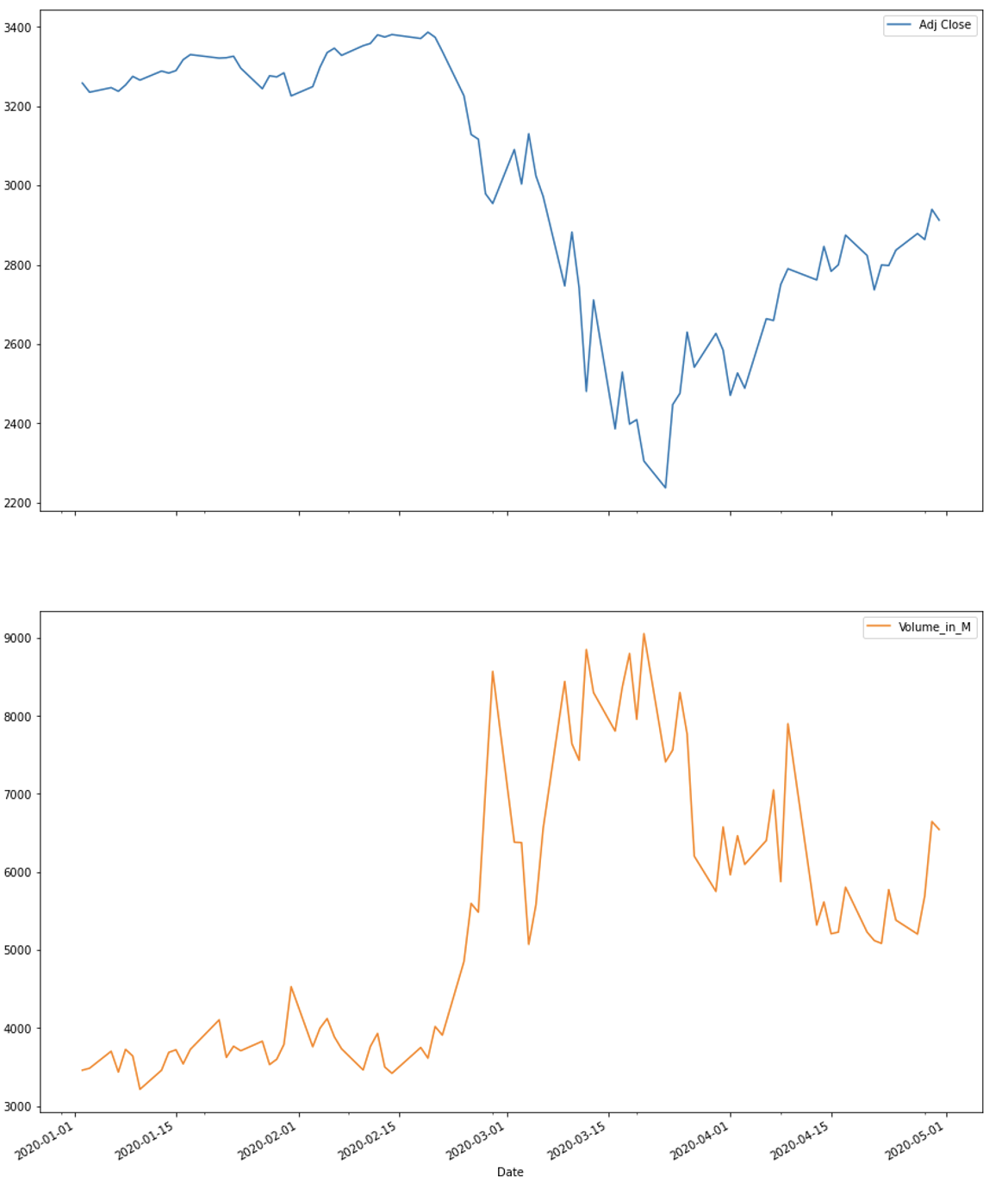2020年3月，由于疫情的蔓延，全球多个国家都开始实行封控政策。从结果可以看出市场对封控政策的反响巨大，但与此同时多地政府也采取了各式各样的经济政策来稳定市场，人们的生产生活在疫情后开启了新的模式。在这里，我们假设疫情的一系列政策会在短时间内持续下去。根据公共信息的评估，大家可以酌情调整模型和假设。在本项目中，我们的目标是使用自2020年2月15日起的历史数据，预测未来1个工作日的调整后闭市指数价格。

## 数据预处理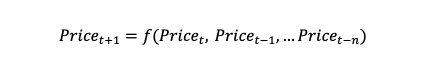• 将时间转换为变量
• 更改价格数据
• 寻找周期和季节性
• 根据周期调整交易量数据

### 1. 将时间转换为变量

```df_mod_forecast = df_mod.reset_index()
df_mod_forecast.info()
```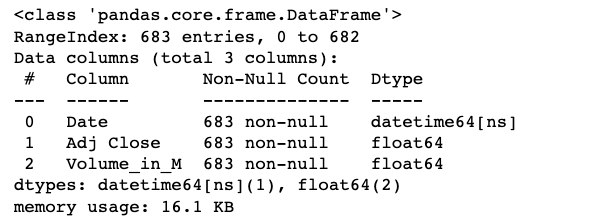```df_mod_forecast.head()
```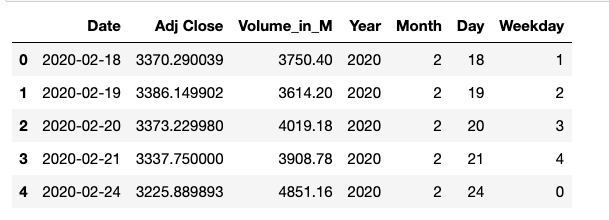### 2. 更改价格数据

```df_mod_forecast['AdjPricePctDelta'] = df_mod_forecast['Adj Close'].pct_change()
```

### 3. 寻找周期和季节性 — 解构时间序列与自相关分析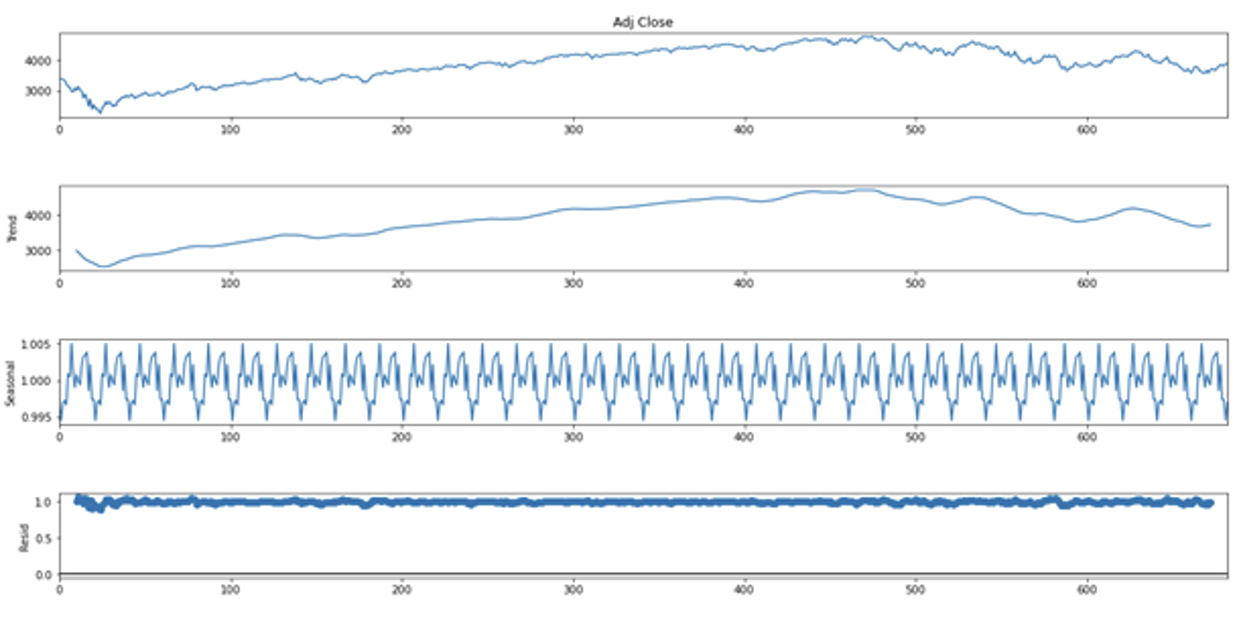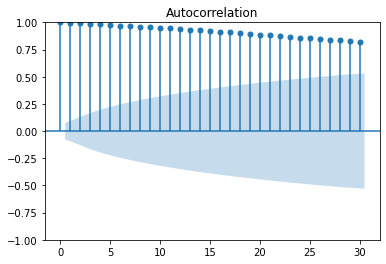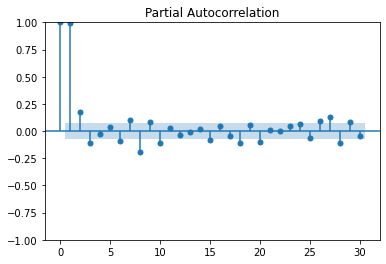ACF显示近30天的历史价格都对当下的价格有影响，而太多的信息会影响模型的效率，甚至带来过拟合的风险。与ACF相比，PACF显示前两至三天价格较为相关，若同时包括t-2和t-1的数据，则模型势必会被t-2与t-1之间的correlation影响。

• 比起价格本身，我们可以从价格变动入手
• 再次印证ARIMA并非该数据的最佳选择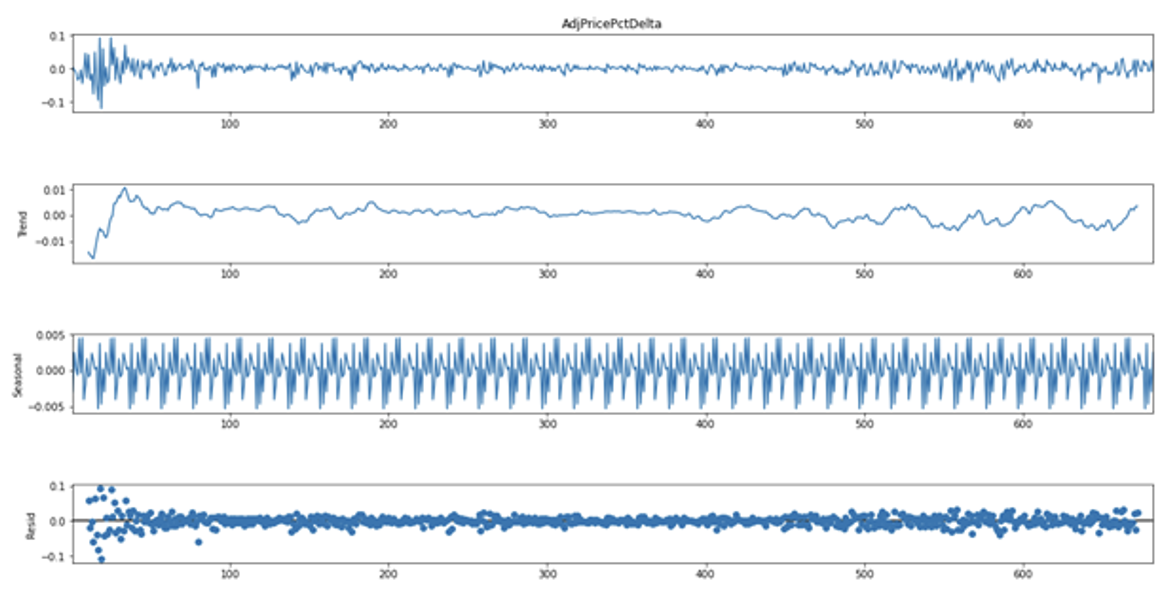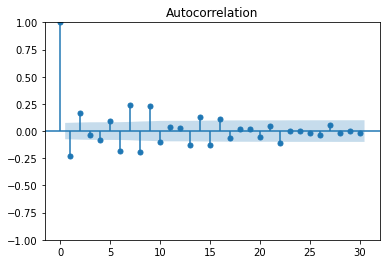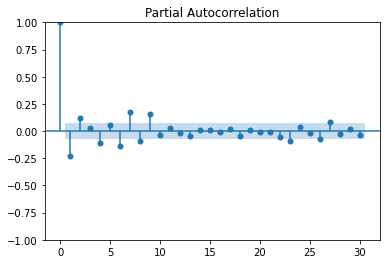```n_seasonal_cycles = math.ceil(df_mod_forecast.shape/20)
df_mod_forecast['seasonality'] = (list(range(0,20))*n_seasonal_cycles[:df_mod_forecast.shape]
```

### 4. 根据周期调整交易量数据

```df_model['t_1_PricePctDelta'] = df_model['AdjPricePctDelta'].shift(periods=1)
```

```df_model['t-1volume'] = df_model['Volume_in_M'].shift(periods=1)
df_model['t-2volume'] = df_model['Volume_in_M'].shift(periods=2)
df_model['t_1_VolumeDelta'] = df_model['t-1volume'] - df_model['t-2volume']
```

```df_model['sign_t_1'] = np.where(df_model['t_1_PricePctDelta']>0,1,0*df_model['t_1_PricePctDelta'])
```